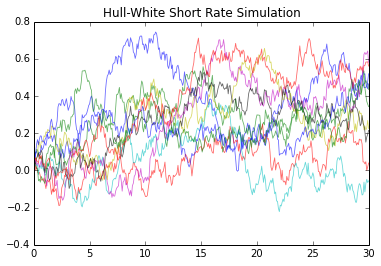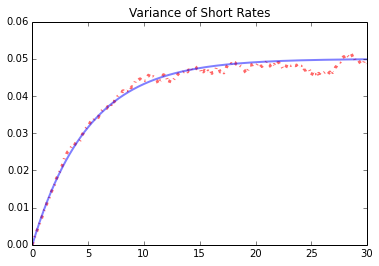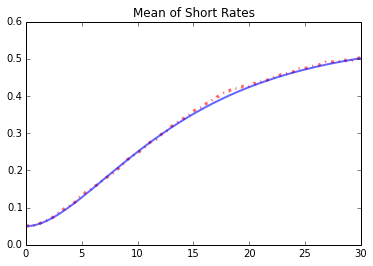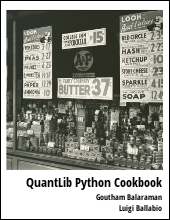Discusses simulation of the Hull White interest rate term structure model in QuantLib Python

Visit here for other QuantLib Python examples. If you found these posts useful, please take a minute by providing some feedback.

The Hull-White Short Rate Model is defined as:

$$dr_t = (\theta(t) - a r_t)dt + \sigma dW_t$$

where $a$ and $\sigma$ are constants, and $\theta(t)$ is chosen in order to fit the input term structure of interest rates. Here we use QuantLib to show how to simulate the Hull-White model and investigate some of the properties.

We import the libraries and set things up as shown below:

In :
import QuantLib as ql
import matplotlib.pyplot as plt
import numpy as np
% matplotlib inline


The constants that we use for this example is all defined as shown below. Variables sigma and a are the constants that define the Hull-White model. In the simulation, we discretize the time span of length 30 years into 360 intervals (one per month) as defined by the timestep variable. For simplicity we will use a constant forward rate term structure as an input. It is straight forward to swap with another term structure here.

In :
sigma = 0.1
a = 0.1
timestep = 360
length = 30 # in years
forward_rate = 0.05
day_count = ql.Thirty360()
todays_date = ql.Date(15, 1, 2015)

In :
ql.Settings.instance().evaluationDate = todays_date

spot_curve = ql.FlatForward(todays_date, ql.QuoteHandle(ql.SimpleQuote(forward_rate)), day_count)
spot_curve_handle = ql.YieldTermStructureHandle(spot_curve)

In :
hw_process = ql.HullWhiteProcess(spot_curve_handle, a, sigma)
rng = ql.GaussianRandomSequenceGenerator(ql.UniformRandomSequenceGenerator(timestep, ql.UniformRandomGenerator()))
seq = ql.GaussianPathGenerator(hw_process, length, timestep, rng, False)


The Hull-White process is constructed by passing the term-structure, a and sigma. To create the path generator, one has to provide a random sequence generator along with other simulation inputs such as timestep and length.

A function to generate paths can be written as shown below:

In :
def generate_paths(num_paths, timestep):
arr = np.zeros((num_paths, timestep+1))
for i in range(num_paths):
sample_path = seq.next()
path = sample_path.value()
time = [path.time(j) for j in range(len(path))]
value = [path[j] for j in range(len(path))]
arr[i, :] = np.array(value)
return np.array(time), arr


The simulation of the short rates look as shown below:

In :
num_paths = 10
time, paths = generate_paths(num_paths, timestep)
for i in range(num_paths):
plt.plot(time, paths[i, :], lw=0.8, alpha=0.6)
plt.title("Hull-White Short Rate Simulation")
plt.show()The short rate $r(t)$ is given a distribution with the properties:

$$E\{r(t) | F_s\} = r(s)e^{-a(t-s)} + \alpha(t) - \alpha(s)e^{-a(t-s)}$$ $$Var\{ r(t) | F_s \} = \frac{\sigma^2}{2a} [1 - e^{-2a(t-s)}]$$ where $$\alpha(t) = f^M(0, t) + \frac{\sigma^2} {2a^2}(1-e^{-at})^2$$

as shown in Brigo & Mercurio's book on Interest Rate Models.

In :
num_paths = 1000
time, paths = generate_paths(num_paths, timestep)


The mean and variance compared between the simulation (red dotted line) and theory (blue line).

In :
vol = [np.var(paths[:, i]) for i in range(timestep+1)]
plt.plot(time, vol, "r-.", lw=3, alpha=0.6)
plt.plot(time,sigma*sigma/(2*a)*(1.0-np.exp(-2.0*a*np.array(time))), "b-", lw=2, alpha=0.5)
plt.title("Variance of Short Rates")

Out:
<matplotlib.text.Text at 0x7f555d561ad0>In :
def alpha(forward, sigma, a, t):
return forward + 0.5* np.power(sigma/a*(1.0 - np.exp(-a*t)), 2)

avg = [np.mean(paths[:, i]) for i in range(timestep+1)]
plt.plot(time, avg, "r-.", lw=3, alpha=0.6)
plt.plot(time,alpha(forward_rate, sigma, a, time), "b-", lw=2, alpha=0.6)
plt.title("Mean of Short Rates")

Out:
<matplotlib.text.Text at 0x7f555d6a9e10>`# Conclusion

This post shows how to simulate Hull-White short rate model using QuantLib Python. You can download the ipython notebook on Hull-White simulations.

quantlib   python   finance

Related PostI am Goutham Balaraman, and I explore topics in quantitative finance, programming, and data science. You can follow me @gsbalaraman.

Checkout my bookUpdated posts from this blog and transcripts of Luigi's screencasts on YouTube is compiled into QuantLib Python Cookbook .